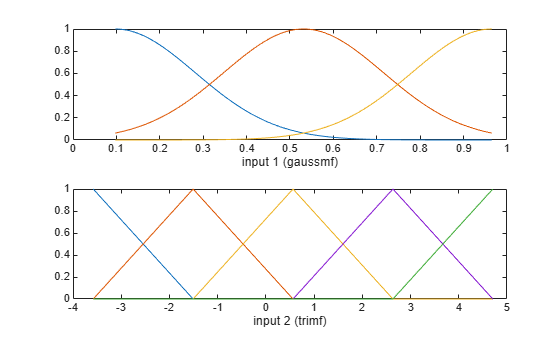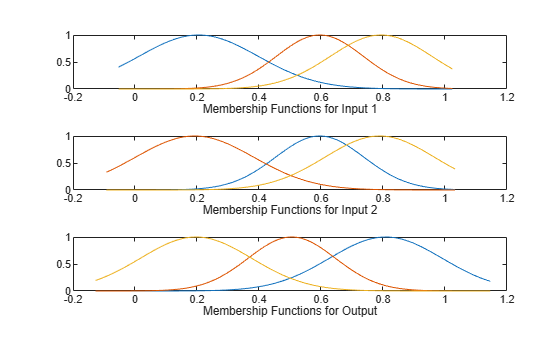# genfis

Generate fuzzy inference system object from data

## Syntax

``fis = genfis(inputData,outputData)``
``fis = genfis(inputData,outputData,options)``

## Description

example

````fis = genfis(inputData,outputData)` returns a single-output Sugeno fuzzy inference system (FIS) using a grid partition of the given input and output data.```

example

````fis = genfis(inputData,outputData,options)` returns a FIS generated using the specified input/output data and the options specified in `options.`. You can generate fuzzy systems using grid partitioning, subtractive clustering, or fuzzy c-means (FCM) clustering.```

## Examples

collapse all

Define training data.

```inputData = [rand(10,1) 10*rand(10,1)-5]; outputData = rand(10,1);```

Generate a fuzzy inference system.

`fis = genfis(inputData,outputData);`

The generated system, `fis`, is created using grid partitioning with default options.

Define training data.

```inputData = [rand(10,1) 10*rand(10,1)-5]; outputData = rand(10,1);```

Create a default `genfisOptions` option set for grid partitioning.

`opt = genfisOptions('GridPartition');`

Specify the following input membership functions for the generated FIS:

• `3` Gaussian membership functions for the first input variable

• `5` triangular membership functions for the second input variable

```opt.NumMembershipFunctions = [3 5]; opt.InputMembershipFunctionType = ["gaussmf" "trimf"];```

Generate the FIS.

`fis = genfis(inputData,outputData,opt);`

Plot the input membership functions. Each input variable has the specified number and type of input membership functions, evenly distributed over their input range.

```[x,mf] = plotmf(fis,'input',1); subplot(2,1,1) plot(x,mf) xlabel('input 1 (gaussmf)') [x,mf] = plotmf(fis,'input',2); subplot(2,1,2) plot(x,mf) xlabel('input 2 (trimf)')```Obtain input and output training data.

```load clusterDemo.dat inputData = clusterDemo(:,1:2); outputData = clusterDemo(:,3);```

Create a `genfisOptions` option set and specify the range of influence for each data dimension. Specify `0.5` and `0.25` as the range of influence for the first and second input variables. Specify `0.3` as the range of influence for the output data.

```opt = genfisOptions('SubtractiveClustering',... 'ClusterInfluenceRange',[0.5 0.25 0.3]);```

Generate the FIS.

`fis = genfis(inputData,outputData,opt);`

The generated FIS contains one rule for each cluster.

`showrule(fis)`
```ans = 3x83 char array '1. If (in1 is in1cluster1) and (in2 is in2cluster1) then (out1 is out1cluster1) (1)' '2. If (in1 is in1cluster2) and (in2 is in2cluster2) then (out1 is out1cluster2) (1)' '3. If (in1 is in1cluster3) and (in2 is in2cluster3) then (out1 is out1cluster3) (1)' ```

Obtain the input and output data.

```load clusterDemo.dat inputData = clusterDemo(:,1:2); outputData = clusterDemo(:,3);```

Create a `genfisOptions` option set for FCM Clustering, specifying a Mamdani FIS type.

`opt = genfisOptions('FCMClustering','FISType','mamdani');`

Specify the number of clusters.

`opt.NumClusters = 3;`

Suppress the display of iteration information to the Command Window.

`opt.Verbose = 0;`

Generate the FIS.

`fis = genfis(inputData,outputData,opt);`

The generated FIS contains one rule for each cluster.

`showrule(fis)`
```ans = 3x83 char array '1. If (in1 is in1cluster1) and (in2 is in2cluster1) then (out1 is out1cluster1) (1)' '2. If (in1 is in1cluster2) and (in2 is in2cluster2) then (out1 is out1cluster2) (1)' '3. If (in1 is in1cluster3) and (in2 is in2cluster3) then (out1 is out1cluster3) (1)' ```

Plot the input and output membership functions.

```[x,mf] = plotmf(fis,'input',1); subplot(3,1,1) plot(x,mf) xlabel('Membership Functions for Input 1') [x,mf] = plotmf(fis,'input',2); subplot(3,1,2) plot(x,mf) xlabel('Membership Functions for Input 2') [x,mf] = plotmf(fis,'output',1); subplot(3,1,3) plot(x,mf) xlabel('Membership Functions for Output')```To create a type-2 FIS from input/output data, you must first create a type-1 FIS using `genfis`.

Load training data and generate a FIS using subtractive clustering.

```load clusterDemo.dat inputData = clusterDemo(:,1:2); outputData = clusterDemo(:,3); opt = genfisOptions('SubtractiveClustering',... 'ClusterInfluenceRange',[0.5 0.25 0.3]); fisT1 = genfis(inputData,outputData,opt); fisT1.Outputs```
```ans = fisvar with properties: Name: "out1" Range: [-0.1274 1.1458] MembershipFunctions: [1x3 fismf] ```

Convert the generated FIS to a type-2 FIS.

`fisT2 = convertToType2(fisT1);`

Since the initial type-1 FIS is a Sugeno system, only the input MFs are converted to type-2 MFs.

## Input Arguments

collapse all

Input data, specified as an N-column array, where N is the number of FIS inputs.

`inputData` and `outputData` must have the same number of rows.

Output data, specified as an M-column array, where M is the number of FIS outputs.

When using grid partitioning, `outputData` must have one column. If you specify more than one column for grid partitioning, `genfis` uses the first column as the output data.

`inputData` and `outputData` must have the same number of rows.

FIS generation options, specified as a `genfisOptions` option set. If you do not specify `options`, `genfis` uses a default grid partitioning option set.

You can generate fuzzy systems using one of the following methods, which you specify when you create the option set:

• Grid partitioning — Generate input membership functions by uniformly partitioning the input variable ranges, and create a single-output Sugeno fuzzy system. The fuzzy rule base contains one rule for each input membership function combination.

`options = genfisOptions('GridPartition');`
• Subtractive clustering — Generate a Sugeno fuzzy system using membership functions and rules derived from data clusters found using subtractive clustering of input and output data. For more information on subtractive clustering, see `subclust`.

`options = genfisOptions('SubtractiveClustering');`
• FCM Clustering — Generate a fuzzy system using membership function and rules derived from data clusters found using FCM clustering of input and output data. For more information on FCM clustering, see `fcm`.

`options = genfisOptions('FCMClustering');`

## Output Arguments

collapse all

Fuzzy inference system, returned as a `mamfis` or `sugfis` object. The properties of `fis` depend on the type of clustering used and the corresponding `options`.

Clustering TypeFuzzy System TypeInput Membership FunctionsFuzzy RulesOutput Membership Functions
Grid PartitioningSugenoEach input variable has evenly distributed input membership function. Specify the number of membership functions using `options.NumMembershipFunctions`. Specify the membership function type using `options.InputMembershipFunctionType`.One rule for each input membership function combination. The consequent of each rule corresponds to a different output membership function.One output membership function for each fuzzy rule. Specify the membership function type using `options.OutputMembershipFunctionType`.
Subtractive ClusteringSugenoEach input variable has one `'gaussmf'` input membership function for each fuzzy cluster.One rule for each fuzzy clusterEach output variable has one `'linear'` output membership function for each fuzzy cluster.
FCM ClusteringMamdani or SugenoEach input variable has one `'gaussmf'` input membership function for each fuzzy cluster.One rule for each fuzzy clusterEach output variable has one output membership function for each fuzzy cluster. The membership function type is `'gaussmf'` for Mamdani systems and `'linear'` for Sugeno systems.

If `fis` is a single-output Sugeno system, you can tune the membership function parameters using the `anfis` function.

Generating a type-2 FIS is not supported by `genfis`. Instead, generating a type-1 FIS and convert it using the `convertToType2` function.

## Version History

Introduced in R2017a

expand all

Warns starting in R2019b